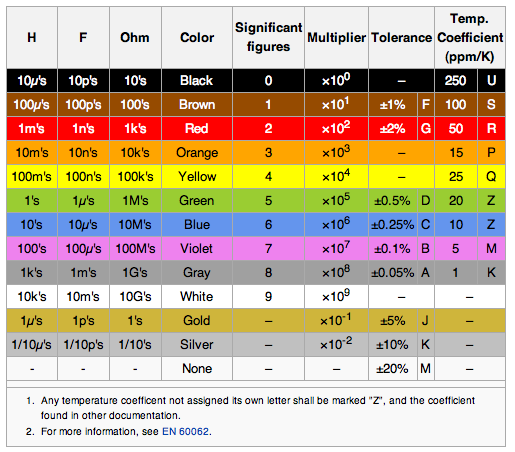Resistance, Resistivity Circuits   electronics   resistors   capacitors ```Resistance of a wire in Ω R = ρL/A ρ is resistivity of the material in Ω-m L is length in meters A is cross-sectional area in m² A = πr², r is radius of wire in m resistivity Cu 17.2e-9 Ω-m conductivity = σ = 1/ρ Conductance = 1/R = A/ρL = Aσ/L Stretched wire If you stretch it so that it is K longer then cross-sectional area goes down by K R goes up by K² If you stretch it so that diameter reduces by K, then area decreases by K², length goes up by K² and R goes up by K⁴ Sheet resistance Rs, in ohms per square, Ω/⃞) is the resistance of a film of material. Rs = resistivity / thickness Resistance of the sheet is R = Rs(L/W) drift velocity Vd Vd = I / nqA I is current n is the number of charge carriers per m³ for copper, 8.5e28 electrons per m³ A is the cross sectional area in m² A = πr², r is radius of wire in m q is the charge of the charge carriers (electrons) q = –1.602e–19 Coulomb (charge on an electron) or Vd = mσΔV / ρεfℓ m is molecular mass of the conductor in kg (mol mass in g / 1000) σ is electric conductivity of the conductor in S/m (Siemens/meter) (1/resistivity in Ω-m) ΔV is the voltage across the conductor ρ is the density in kg/m³ ε is the elementary charge, in C (1.602e–19 Coulomb) f is the number of free electrons per atom ℓ is the length of the conductor, in m Power P = E*I P = E²/R P = I²R 1HP = 746 watts 1 amp = 1 coulomb per second = 6.242e18 electrons per sec Ohm's law For constant R and varying current, E = IR, E and I are proportional For constant R and varying voltage, I = E/R, E and I are proportional For constant voltage and varying R, I = E/R, current is inversely proportional to resistance. For constant voltage and varying current, R = E/I, resistance is inversely proportional to current. For constant current and varying R, E = IR, voltage is proportional to resistance For constant current and varying voltage, R = E/I, resistance is proportional to voltage Temperature Change in Resistance Rt = Ro(1 + α∆T) α is temperature coef in /ºC R = L/Aσ σ = L/AR σ is conductivity in 1/Ω-m α for copper is 0.0039 /ºC α for Aluminum is 0.0039 /ºC α for nickel is 0.006 /ºC α for iron is 0.005 /ºC α for silver is 0.0038 /ºC α for Tungston is 0.0045 /ºC α for Zinc is 0.0037 /ºC α for tin is 0.0045 /ºC α for nichrome is 0.0004 /ºC α for Carbon (amorphous) is –0.0005 /ºC α for mercury is 0.0009 /ºC α for Constantan is 0.000008 /ºC α for Manganin is 0.000002 /ºC Volume Resistivity resistivity Ag 15.9e-9 Ω-m resistivity Cu 17.2e-9 Ω-m or 17.2e-6 ohm-mm resistivity Au 22.14e-9 Ω-m resistivity Al 28.2e-9 Ω-m resistivity brass 35e-9 Ω-m resistivity W 56e-9 Ω-m resistivity Zn 68e-9 Ω-m resistivity Ni 69e-9 Ω-m resistivity Fe 100e-9 Ω-m resistivity Chromium 125e-9 Ω-m resistivity Steel (cast) 160e-9 Ω-m resistivity Pt 105e-9 Ω-m resistivity Pb 208e-9 Ω-m resistivity Hg 961e-9 Ω·m resistivity Stainless steel (304) 750e-9 Ω-m resistivity constantan 500e-9 Ω-m resistivity Nichrome 1500e-9 Ω-m resistivity carbon 6–40 µΩm resistivity silicon 640–1000 Ωm resistivity germanium 0.5-1 Ωm Resistivity of pure water is 182 kΩ·m Resistivity of sea water is 0.2 Ω·m density resistivity (resistivity x density) Al 28.2e-9 Ω-m * 2700 kg/m³ = 76e-6 Ω-kg/m² Cu 17.2e-9 Ω-m * 8960 kg/m³ = 154e-6 Ω-kg/m² Ag 15.9e-9 Ω-m * 10500 kg/m³ = 167e-6 Ω-kg/m² Au 22.14e-9 Ω-m * 19300 kg/m³ = 427e-6 Ω-kg/m² Zn 68e-9 Ω-m * 7130 kg/m³ = 485-6 Ω-kg/m² Fe 100e-9 Ω-m * 7870 kg/m³ = 787e-6 Ω-kg/m² W 56e-9 Ω-m * 19300 kg/m³ = 1080e-6 Ω-kg/m² Pt 105e-9 Ω-m * 21500 kg/m³ = 2257-6 Ω-kg/m² Color Codes 0 Black 1 Brown 2 Red 3 Orange 4 Yellow 5 Green 6 Blue 7 Violet 8 Gray 9 White 5% Gold x0.1 10% Silver x0.01 K (silver) 10% J (gold) 5% F (brown) 1% G (red) 2% D (green) 0.5% C (blue) 0.25% B (violet) 0.1% (grey) 0.05% Standard values 10% 10, 12, 15, 18, 22, 27, 33, 39, 47, 56, 68, 82, 100 and multiples of these values Standard values 5% 10, 11, 12, 13, 15, 16, 18, 20, 22, 24, 27, 30, 33, 36, 39, 43, 47, 51, 56, 62, 68, 75, 82, 91, 100 ```Home Area, Volume Atomic Mass Black Body Radiation Boolean Algebra Calculus Capacitor Center of Mass Carnot Cycle Charge Chemistry   Elements   Reactions Circuits Complex numbers Constants Curves, lines deciBell Density Electronics Elements Flow in fluids Fourier's Law Gases Gravitation Greek Alphabet Horizon Distance Interest Magnetics Math   Trig Math, complex Maxwell's Eq's Motion Newton's Laws Octal/Hex Codes Orbital Mechanics Particles Parts, Analog IC   Digital IC   Discrete Pendulum Planets Pressure Prime Numbers Questions Radiation Refraction Relativistic Motion Resistance, Resistivity Rotation Series SI (metric) prefixes Skin Effect Specific Heat Springs Stellar magnitude Thermal Thermal Conductivity Thermal Expansion Thermodynamics Trigonometry Units, Conversions Vectors Volume, Area Water Wave Motion Wire, Cu   Al   metric Young's Modulus